## MS Math – Week of Oct 25-286th Grade Math – Mrs. Evans

This week we will finish Chapter 2 with a test on Tuesday.   This test focuses, largely, on multiplying and dividing fractions and mixed numbers.  For students who need additional help or practice with these skills, please contact Mrs. Evan s or go to IXL.COM – sections H and I.

Beginning on Wednesday we will be learning about how we can represent real world quantities as negative integers and rational numbers.  It is useful to be able to represent every situation as either positive or negative in order to think of them in a mathematical way.  We will spend time making sure that we can order positive and negative rational numbers properly before we put them into our tool box for all our future use!

Math Grade Math – Mrs. Evans and Mrs. VonFeldt

This week we will finish Module 3, which is also the conclusion of Unit 1.  Upon completion of this unit, students will have the ability to use rational numbers (fractions, decimals and integers) that are both negative and positive, in all the basic operations.  They will have practiced, in lesson 3.6, this week, to apply a strategy that will help them in solving real world problems that deal with both decimals and fractions. The Unit 1 Test will be this Friday. For any students who feel the need for additional practice on the skills we have learned in Unit 1, please go to IXL.COM – section H.

8th Grade Math – Mrs. Ernest

Students have been learning how to graph from an equation or a data table. This week we will be expanding our knowledge of linear equations, functions in general and the coordinate plane.

https://www.cuemath.com/algebra/linear-equations/

Algebra – Mrs. VonFeldt

We are continuing to explore the question: How can we use linear relationships to solve problems? A graph is one representation of a function. This week, students will learn to graph linear equations using two different strategies: 1) make a table and 2) graph using intercepts. If the x and y-intercepts are known, or can be easily found, then a straight line going through those points makes a line: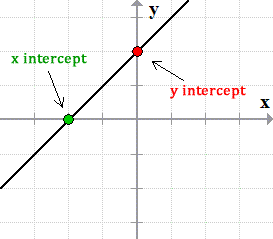We will also begin exploring slope. What is slope and how can you calculate it between two points? This will lead us into the slope-intercept form next week.## MS Math – October 18-226th Grade Math – Mrs. Evans

This week we will be dividing fractions and mixed numbers.  Dividing fractions is actually a pretty simple thing to do, especially after we know how to multiply fractions.  This is because a division can be considered the same as multiplying by the reciprocal.  A reciprocal is just the “flip” of a number or fraction.  For example the reciprocal of 3/5 is 5/3.  The numerators and denominators just trade places.  We have a great way to remember how to divide by fractions:When dividing with a mixed number, just as we do in multiplying, we switch the mixed number into an improper fraction and then continue as if it were any other fraction.  Here is a reminder for those who need help converting a mixed number into an improper fraction: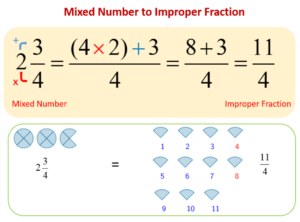We will practice being able to apply our new skills in problem solving as we collaborate with each other.  We will also focus on being able to verbalize how to multiply and divide fractions.  We will finish the week with a KAHOOT covering all the topics from Chapter 2.

Math Grade Math – Mrs. Evans and Mrs. VonFeldt

This week we will continue working in Module 3, not only adding and subtracting positive and negative rational numbers but multiplying and dividing them.  These are foundational skills requiring a degree of fluency.  Students who are struggling with these skills should seek help so that they are confident as the move into algebra later in the year.  A great place for extra help with immediate feedback on how a student is doing, is http://IXL.COM

The rules we learned with integers that help us to multiply and divide are still applicable with rational numbers.  Here are the rules to remind us:8th Grade Math – Mrs. Ernest

This week students will be refining their graphing skills, practice finding the difference between points, and interpret graphs through word problems. Students struggling with graphing skills should revisit these IXL activities:Algebra – Mrs. VonFeldt

This week, we will finish up Chapter 2 with a test on Tuesday. At the end of the week, students will study a roller coaster problem. They will take data, make a table of values and a graph to evaluate their results and determine if the coaster is safe for tall riders. This will introduce linear functions and graphs, which will be the focus of Chapter 3.

## MS Math – Oct 11-15, 20216th Grade Math – Mrs. Evans

This week we will be extending our understanding and skills with fraction operations, beginning with writing decimals as fractions and fractions with decimals.  We will compare and order decimals and fractions and then we will begin to multiply fractions, learning a wonderful trick to simplify them as we multiply.  Here is a little picture to help students, and families, convert between decimals and fractions:Converting from a decimal to a fraction is done by remembering that a decimal is a portion of a factor of ten, as shown here:We understand that we can write a decimal as a fraction and a fraction as a decimal simply by remembering these simple lessons.  Here is a video that can help students who want a little more help converting from a decimal to a fraction and a fraction to a decimal:

Math Grade Math – Mrs. Evans and Mrs. VonFeldt

This week we will be working in Module 3, in which we will apply all that we learned in Modules 1 and 2 to rational numbers.  Rational numbers are any number that can be represented by a ratio of two integers (the second of which is not zero).  The most important thing to know about rational numbers is that they include decimals and fractions.  Here is a picture showing what rational numbers are:We apply the rules and logic we learned about operations with negatives to decimals and fractions.  This means we will be able to do all the basic operations to all the types of numbers!    For any students who want to review how to convert between decimals and fractions, please see the video links above (6th grade math update).

8th Grade Math – Mrs. Ernest

This week 8th graders are reviewing algebra concepts from last week and applying these ideas to graphing problems. We will be working through Chapter 2 this week, focusing on how to graph, how to graph linear equations from an x,y table and writing linear equations from word problems.

Graphing Review:

Algebra – Mrs. VonFeldt

This week, students will continue to solve and master solving one-variable equations. They will learn how to solve proportional relationships using cross products: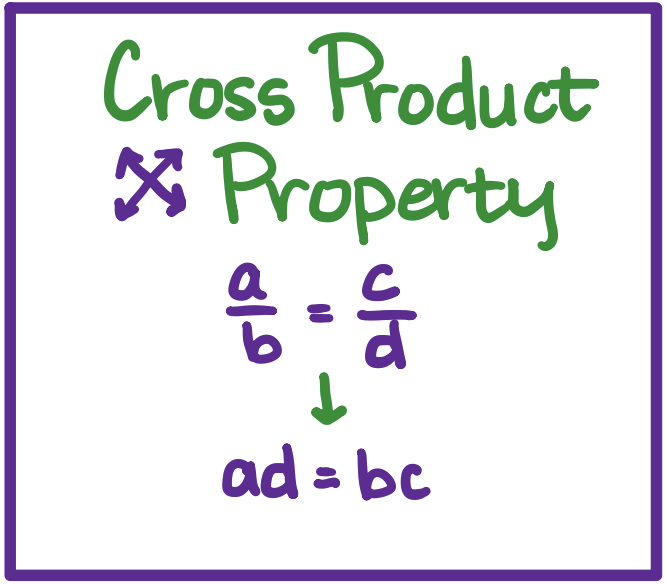We will also learn how to rewrite equations and formulas to be solved for a specific variable. This is often helpful to rewrite formulas with multiple variable inputs. At the end of the week, we will wrap up Chapter 2 with a team test on Thursday. The individual test will be next Tuesday.

## MS Math – Oct 4-8, 2021

1.6th Grade Math – Mrs. Evans

This week we will finish Chapter 1 with a test on Wednesday.   We will carry with us the ability to do all decimal arithmetic!  For help with decimal arithmetic, see the link to the Math Antics video in last week’s post.   Also in this chapter we learned about finding the least common multiple (LCM) and greatest common factor (GCF) and how to use these values to solve real world problems.  One of the important properties that we have reviewed in this chapter is the distributive property.  This is a property that we will use a great deal in algebra.  The distributive property allows us to distribute a factor across a sum.  Here is how it looks: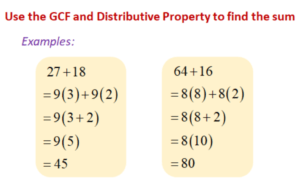After we finish with the Chapter 1 test, we will continue to learn more about decimals in Chapter 2.

Math Grade Math – Mrs. Evans and Mrs. VonFeldt

This week we will review and test on Module 2 which covers multiplying and dividing integers.  If we remember the rules (shown in last week’s post), it can be a straight forward process.  It is, however, necessary that we continue to ALWAYS use the proper order of operations.  Here is a reminder of PEMDAS which helps us keep  the order correct: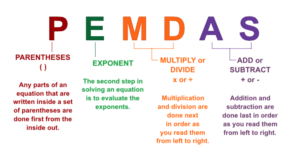For students who need help with Module 2 concepts, here is a video summarizing how we multiply and divide integers.

When we finish with the Module 2 test, we will begin to apply what we have learned about negatives to rational numbers (fractions, decimals and mixed numbers).

8th Grade Math – Mrs. Ernest

This week students will begin solving multi-step algebraic equations. Students will be working through the remainder of Chapter 1, and we will begin Chapter 2 by the end of this week. I will be specifically looking for each student to write out their work as clearly as possible! See the image below for an example one-step problem and the requirements for showing work on homework.Algebra – Mrs. VonFeldt

This week is all about solving linear equations. By the end of the week, students should be able to solve all types of one variable equations using the following steps:

• Distribute;
• Combine like terms;